# Gaussian Scatter Model

This knowledge base article will describe how to create a Gaussian scatter distribution using FREDs Scripted BSDF scatter model. This distribution is commonly used for simulating diffuser elements.

A scripted scatter model type can be created by one of the following methods:

1. Menu > Create > New Scatter Model
2.  Toolbar button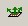3. Right mouse click on the Scatterers folder and select Create a New Scatterer

Once the Scatter dialog has been opened, the Scripted (BSDF given by user-script) type should be selected.  The scripted scatter model is defined in direction cosine space using the incident ray, specular ray, and scatter ray direction vectors as shown below: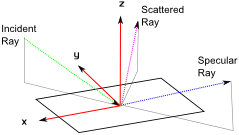The definition of the Gaussian scatter distribution in this model uses the following form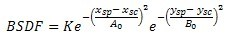The normalization factor K is given by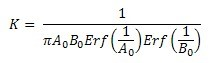In this construction A 0 and B0 are related to the FWHM of the distribution in the x and y directions, xsp and xsc are the X specular and scatter direction components, and ysp and ysc are the Y specular and scatter direction components. The normalization factor is included so that the total integrated scatter (TIS) is 100% at normal incidence.

For the Gaussian distribution we can relate the parameters A 0 and B0 to the FWHM of the BSDF by the equations: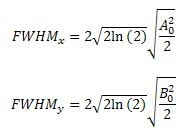In the editor window for the scripted scatter model the comments header provides information regarding the available input and output variables for use in the BSDF definition. This scripted Gaussian scatter model will make use only of g_Xspec, g_Xscat, g_Yspec, g_Yscat and g_bsdf, corresponding to xsp, xsc, ysp, ysc, and BSDF as defined above.

The subroutine for the scripted scatter model is shown below:

```Sub EvalScatter( ByVal g_Xinc#, ByVal g_Yinc#, ByVal g_Zinc#, ByVal g_Xspec#, ByVal g_Yspec#, ByVal g_Zspec#, ByVal g_Xscat#, ByVal g_Yscat#, ByVal g_Zscat#, ByVal g_ran#, ByVal g_TISrequest As Boolean, ByRef g_Xpos#, ByRef g_Ypos#, ByRef g_Zpos#, ByRef g_w#, ByRef g_frac#, ByRef g_numA&, ByRef g_numB&, ByRef g_bsdf# )
Dim K As Double
Dim A0 As Double, B0 As Double, delA As Double, delB As Double

'FWHM related parameters

If Not g_TISrequest Then

delA = (g_Xspec - g_Xscat)/A0
delB = (g_Yspec - g_Yscat)/B0
'normalization constant
K = PI*A0*B0*Erff(1/A0)*Erff(1/B0)

g_bsdf = Exp(-delA*delA)*Exp(-delB*delB)/K

Else

g_Xpos = 0 : g_Ypos = 0 : g_Zpos = 0 : g_frac = 1 : g_w = 0.5
g_numA = 101 : g_numB = 101

End If

End Sub
```

For the values A 0 = 30 degrees and B0 = 5 degrees, we calculate using the equations above that FWHMx = 49.95o and FWHMy = 8.33o. A model with a single ray incident on a scattering surface with this scripted scatter BSDF was raytraced to produce the following 3D BSDF plot in FRED.  The TIS at normal incidence is reported to be 99.956%, as expected due to the normalization factor.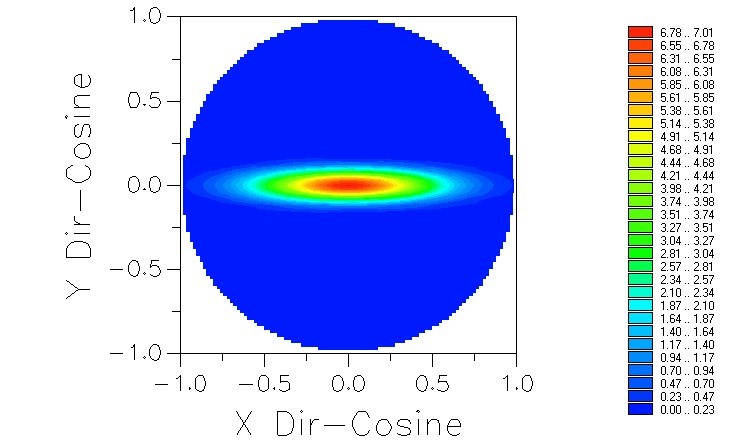One consequence of using the scripted scatter model is that the scatter directions are defined in global coordinates, rather than local to the surface, and so in this case the Gaussian scatter would not rotate as the surface rotates. If instead it is desirable to define the scatter in local coordinates then we can use the extended scripted scatter model which is based on local coordinates and optionally can include polarization effects. Only small modifications to the script are required to append the "t." variables:

```Sub EvalScatter( ByRef t As T_SCATTERSCRIPTEX )

'Sub EvalScatter( ByVal g_Xinc#, ByVal g_Yinc#, ByVal g_Zinc#, ByVal g_Xspec#, ByVal g_Yspec#, ByVal g_Zspec#, ByVal g_Xscat#, ByVal g_Yscat#, ByVal g_Zscat#, ByVal g_ran#, ByVal g_TISrequest As Boolean, ByRef g_Xpos#, ByRef g_Ypos#, ByRef g_Zpos#, ByRef g_w#, ByRef g_frac#, ByRef g_numA&, ByRef g_numB&, ByRef g_bsdf# )
Dim K As Double
Dim A0 As Double, B0 As Double, delA As Double, delB As Double

'FWHM related parameters

If Not g_TISrequest Then

delA = (t.g_Xspec - t.g_Xscat)/A0
delB = (t.g_Yspec - t.g_Yscat)/B0
'normalization constant
K = PI*A0*B0*Erff(1/A0)*Erff(1/B0)

t.g_BSDF = Exp(-delA*delA)*Exp(-delB*delB)/K

Else

t.g_Xpos = 0 : t.g_Ypos = 0 : t.g_Zpos = 0 : t.g_frac = 1 : t.g_w = 0.5
t.g_numA = 101 : t.g_numB = 101

End If

End Sub
```

Example FRED file: gaussianScatterModel.frd